Home > ACC7 > Chapter cc310 > Lesson cc310.1.1 > Problem10-17

10-17.
1. Solve the following equations for the given variable. Homework Help ✎

1. 3x + (−4) = 2y + 9 for y

2. 12 =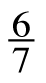x for x

3.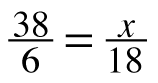for x

4.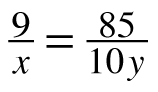for y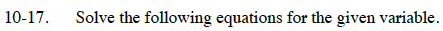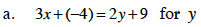3x + (−4) = 2y + 9
−9 −9

$\frac{3x}{2}-\frac{13}{2}=\frac{2y}{2}$

1.5x − 6.5 = y

Isolate the variable.

$\text{Multiply both sides of the equation by }\frac{7}{6}.$

$\left(\frac{7}{6}\right)12=\frac{6}{7}x\left(\frac{7}{6}\right)$

$x=\frac{7}{6}\left(12\right)=14$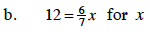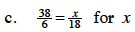You can use cross multiplication to solve this equation.

38 (18) = 6 (x)

$x=\frac{684}{6}=114$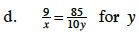See part (c).